Test 2 Section 4 number 26 please!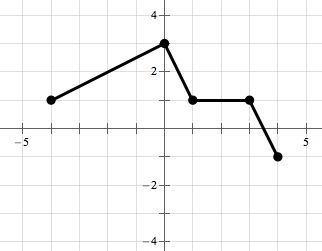There’s my rendering of the figure. Let’s label the points we have.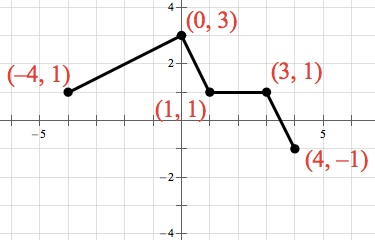The question asks you to identify whether a few different values of f(x) are equal to 1. There are a bunch of times when the f(x) graph equals 1, shown in green below:Remember this trick about converting function notation to points on a graph. If f(a) = b, then (ab) is on the graph of the function f. So when the question asks you whether, and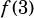equal 1, you should check to see that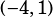,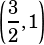, andare all on the graph. Those points are in blue below.All three points the question asks about are on the graph, so the answer is D) I, II, and III.